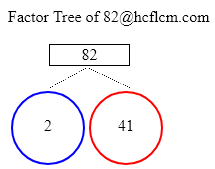# Factors of 82 | Find the Factors of 82 by Factoring Calculator

Factoring Calculator calculates the factors and factor pairs of positive integers.

Factors of 82 can be calculated quickly with the help of Factoring Calculator i.e. 1, 2, 41, 82 positive integers that divide 82 without a remainder.

Factors of 82 are 1, 2, 41, 82. There are 4 integers that are factors of 82. The biggest factor of 82 is 82.

Factors of:

### Factor Tree of 82 to Calculate the Factors

 82 2 41Factors of 82 are 1, 2, 41, 82. There are 4 integers that are factors of 82. The biggest factor of 82 is 82.

Positive integers that divides 82 without a remainder are listed below.

• 1
• 2
• 41
• 82

• 1 × 82 = 82
• 2 × 41 = 82
• 41 × 2 = 82
• 82 × 1 = 82

### Factors of 82 Table

FactorFactor Number
1one
2two
41forty one
82eighty two

### How to find Factors of 82?

As we know factors of 82 are all the numbers that can exactly divide the number 82 simply divide 82 by all the numbers up to 82 to see the ones that result in zero remainders. Numbers that divide without remainder are factors and in this case below are the factors

1, 2, 41, 82 are the factors and all of them can exactly divide number 82.

### Frequently Asked Questions on Factors of 82

1. What are the factors of 82?

Answer: Factors of 82 are the numbers that leave a remainder zero. The ones that can divide 82 exactly i.e. factors are 1, 2, 41, 82.

2.What are Factor Pairs of 82?

Answer:Factor Pairs of 82 are

• 1 × 82 = 82
• 2 × 41 = 82
• 41 × 2 = 82
• 82 × 1 = 82

3. What is meant by Factor Pairs?

Answer:Factor Pairs are numbers that when multiplied together will result in a given product.